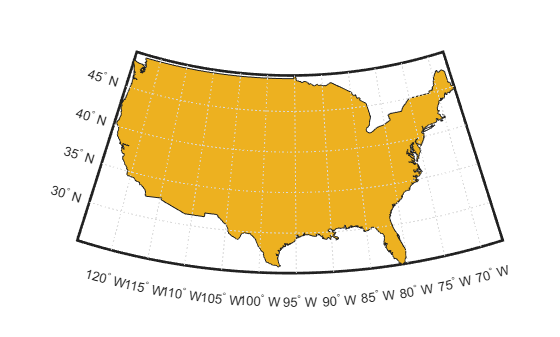# areaint

Surface area of polygon on sphere or ellipsoid

## Syntax

```area = areaint(lat,lon) area = areaint(lat,lon,ellipsoid) area = areaint(lat,lon,units) area = areaint(lat,lon,ellipsoid,units) ```

## Description

`area = areaint(lat,lon)` calculates the spherical surface area of the polygon specified by the input vectors `lat` and `lon`. The calculation uses a line integral approach. The output, `area`, is the fraction of surface area covered by the polygon on a unit sphere. To supply multiple polygons, separate the polygons by NaNs in the input vectors. Accuracy of the integration method is inversely proportional to the distance between lat/lon points.

`area = areaint(lat,lon,ellipsoid)` calculates the surface area of the polygon on the ellipsoid or sphere defined by the input `ellipsoid`, which can be a `referenceSphere`, `referenceEllipsoid`, or `oblateSpheroid` object, or a vector of the form ```[semimajor_axis eccentricity]```. The output, `area`, is in squares units corresponding to the units of `ellipsoid`.

`area = areaint(lat,lon,units)` uses the units defined by `units`, the string scalar or character vector `'degrees'` or `'radians'`. If omitted, default units of degrees are assumed.

`area = areaint(lat,lon,ellipsoid,units)` uses both the inputs `ellipsoid` and `units` in the calculation.

## Examples

collapse all

Consider the area enclosed by a 30º lune from pole to pole and bounded by the prime meridian and 30ºE. You can use the function `areaquad` to get an exact solution:

`area = areaquad(90,0,-90,30)`
```area = 0.0833```

This is 1/12 the spherical area. The more points used to define this polygon, the more integration steps `areaint` takes, improving the estimate. This first attempt takes a point every 30º of latitude:

```lats = [-90:30:90,60:-30:-60]'; lons = [zeros(1,7), 30*ones(1,5)]'; area = areaint(lats,lons)```
```area = 0.0792```

Now, calculate a better estimate, with one point every 1º of latitude:

```lats = [-90:1:90,89:-1:-89]'; lons = [zeros(1,181), 30*ones(1,179)]'; area = areaint(lats,lons) ```
```area = 0.0833```

Load the latitude and longitude coordinates of the perimeter of the conterminous United States. Display the coordinates on a map as a polygon.

```load('conus.mat','uslat','uslon') figure usamap([min(uslat) max(uslat)],[min(uslon) max(uslon)]) geoshow(uslat,uslon,'DisplayType','polygon')```Calculate the area of the conterminous United States in square kilometers. In this case, the `areaint` function returns three areas. The largest area is for the polygon representing the conterminous United States. The other two areas are for the polygons representing Long Island and Martha's Vineyard.

```rKilometers = earthRadius('km'); a = areaint(uslat,uslon,rKilometers)```
```a = 3×1 106 × 7.9256 0.0035 0.0004 ```

Load the latitude and longitude coordinates of the perimeter of the Great Lakes. Display the coordinates on a map as a polygon.

```load('conus.mat','gtlakelat','gtlakelon') figure usamap([min(gtlakelat) max(gtlakelat)],[min(gtlakelon) max(gtlakelon)]) geoshow(gtlakelat,gtlakelon,'DisplayType','polygon')```Calculate the areas of the Great Lakes in square miles. In this case, the `areaint` function returns three areas. The largest area is for the polygon representing Lake Superior, Lake Michigan, and Lake Huron. The other two areas are for the polygons representing Lake Erie and Lake Ontario.

```rMiles = earthRadius('mi'); area = areaint(gtlakelat,gtlakelon,rMiles)```
```area = 3×1 104 × 8.0120 1.0382 0.7634 ```

## Tips

Regardless of the polygon vertex order, the values returned by `areaint` are positive.

## Algorithms

This function enables the measurement of areas enclosed by arbitrary polygons. This is a numerical estimate, using a line integral based on Green's Theorem. As such, it is limited by the accuracy and resolution of the input data.Given sufficient data, the `areaint` function is the best method for determining the areas of complex polygons, such as continents, cloud cover, and other natural or derived features. The calculations in this function employ a spherical Earth assumption. For nonspherical ellipsoids, the latitude data is converted to the auxiliary authalic sphere.

### Topics

Introduced before R2006a

## SupportGet trial now